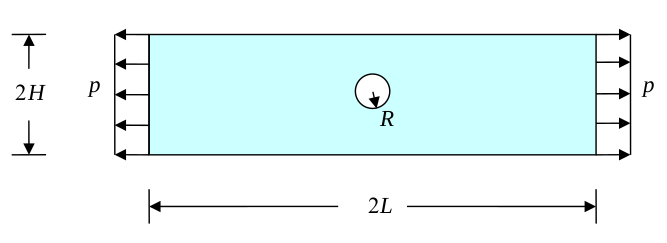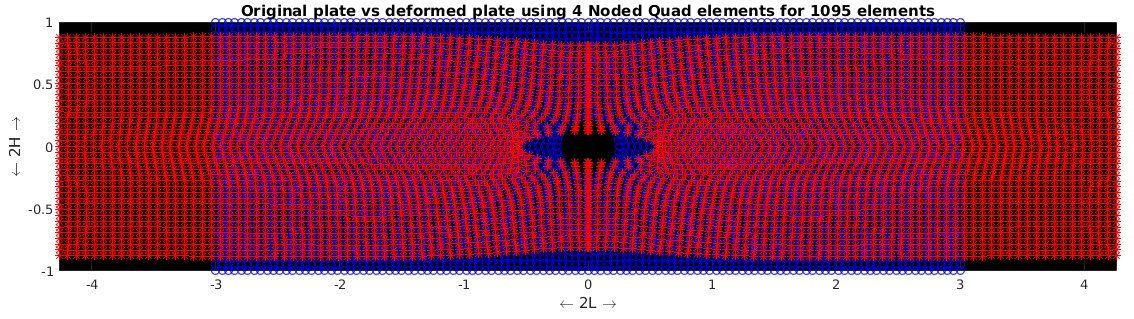# Finite Element Analysis of a plate with a hole using Constant Strain triangle, four and eight noded isoparametric quadrilateral elements

This project aims at studying the deformation of a thin plate with a central circular hole when the plate is loaded in tension with a constant load. Finite element codes were developed in MATLAB using constant strain triangle elements, four- and eight-noded isoparametric elements. A convergence study was performed based on the energy norm a for all three cases and the stress concentration factor around the circular hole was also investigated.The computed results were then compared with the results obtained using commercial code ABAQUS.Problem setupSimulated deformationError in energy norm